# How to Calculate Number of Days in Microsoft Excel

In this article we will learn about how we can calculate the number of days in Microsoft Excel 2010 and Microsoft Excel 2013.

Often we need to calculate the number of days between two dates.

If these dates get frequently changed then a formula is required to avoid repetitive work.

Let us take an example:

• Consider Today is 1-Aug-14
• If 1-Jan is first & 2-Jan is second day of the year,then find out Today’s Day of the current year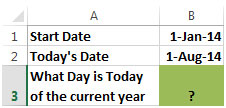• Subtract Start Date from Today’s Date & then add 1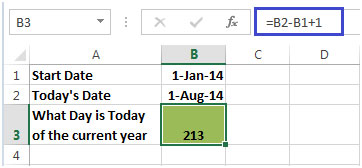Take another example:

Column A& B contain Start & End Date and Column C contains Interval Unit. In column D, output is required on the basis of Interval Unit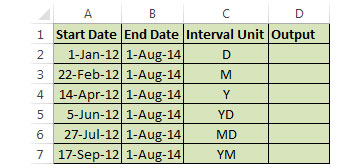In this example, we will use Datedif Function. This function can calculate the number of days between two Dates.

Datedif function is used to calculate interval between two dates in days, months or years.

Syntax =DATEDIF(start_date, end_date, interval_unit)

Datedif Function has 3 arguments:

• start_date: The starting date
• end_date: The last date
• interval_unit: Tells the function to find the number of days ("D"), complete months ("M"), or complete years ("Y") between the two dates.
• To calculate Number of Days,enter the formula in Cell D2=DATEDIF(A2,B2,C2)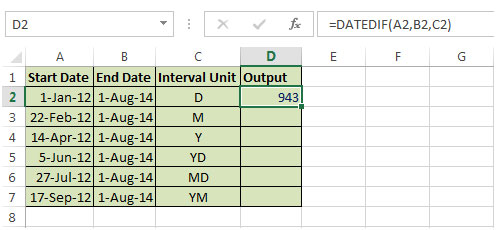• To calculate Number of Months,enter the formulain Cell D3=DATEDIF(A3,B3,C3)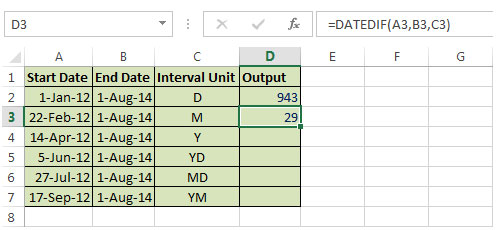• To calculate Number of Years,enter the formula in Cell D4=DATEDIF(A4,B4,C4)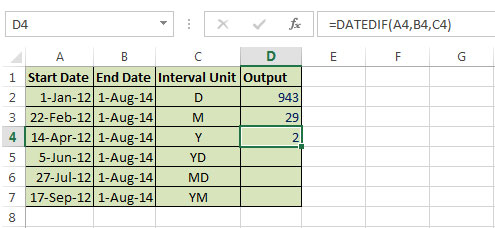• To calculate Number of Days after current year,enter the formula in Cell D5=DATEDIF(A5,B5,C5)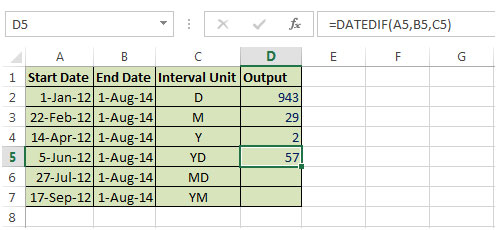• To calculate Number of Days after current Month,enter the formula in Cell D6=DATEDIF(A6,B6,C6)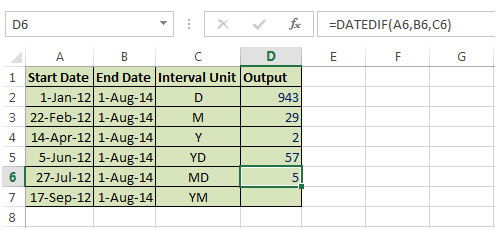• To calculate the Number of Month after current year,enter the formula in Cell D7=DATEDIF(A7,B7,C7)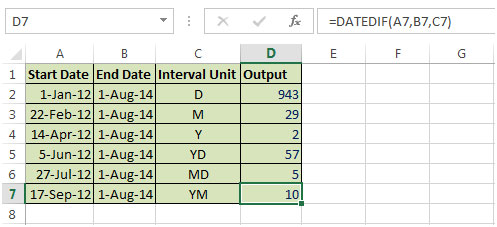1.